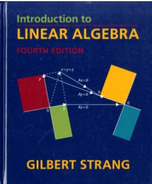Introduction To Linear Algebra - 4 Edition - Chapter 3.5 - Problem 45
Register Now

Join StudySoup

Get Full Access to Introduction To Linear Algebra - 4 Edition - Chapter 3.5 - Problem 459780980232714

# Inside Rn, suppose dimension (V) + dimension (W) > n. Show that some nonzero vector is

Introduction to Linear Algebra | 4th Edition

Problem 45

Inside Rn, suppose dimension (V) + dimension (W) > n. Show that some nonzero vector is in both V and W.

Accepted Solution
Step-by-Step Solution:
Step 1 of 3

Lecture 14: Basic Rules of Diﬀerentiation Polynomials and Exponentials (Section 3.2) Derivative of a Constant d If c is a constant, the(c)= dx ✻ ✛ ✲ ❄ Power functions of the form f(x)= x n d 1) (x)= dx ✻ ✛ ✲ ❄

###### Chapter 3.5, Problem 45 is Solved

Step 2 of 3

Step 3 of 3

Unlock Textbook Solution